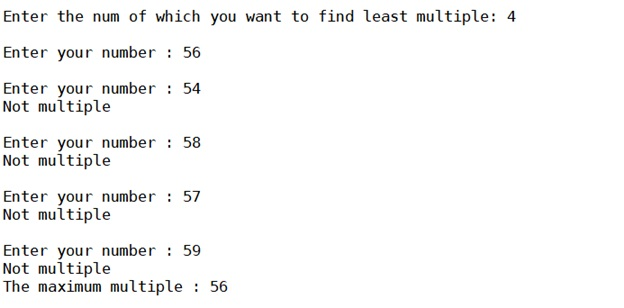# Python program to find the least multiple from given N numbers

Here, we are going to find the least multiple from the given N numbers using Python program.
Submitted by Anuj Singh, on September 14, 2019

Here, we will be framing code for finding the least multiple of a number x from a given set of numbers (set of 5 numbers in this program, and it could be many numbers as per the problem).

There will be a problem while you go to find the least multiple.

There are many ways of doing this but this time, we have to thought of most computationally efficient algorithm to do so.

Using for loop and checking every time might be the thing which works better than other approached. But it about checkings of the least value to be considered for comparing.

So let's work it out.

Following is the code for such problem,

```n = 0

num = 0

minnum = 13
j = 0
x = int(input("Enter the num of which you want to find least multiple: "))
while n<5:
num = int(input("Enter your number : "))
if num%x == 0:
j = j + 14
if j == 14:
minnum = num
if num < minnum:
minnum = num
else:
print("Not multiple")

n += 1
if minnum%x == 0:
print("The maximum multiple :",minnum)
else:
print("No multiple there")
```

OutputPreparation